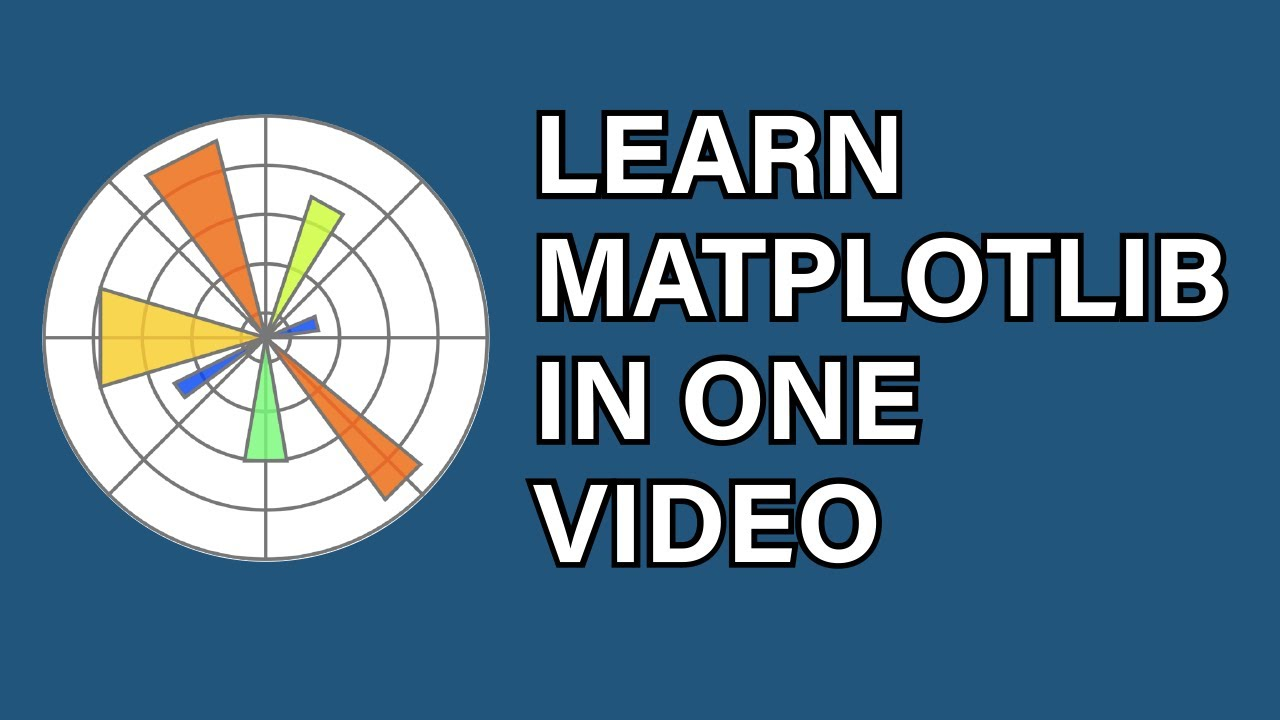# Learn Matplotlib in one Video - Matplotlib Tutorial 2020This video provides the most complete coverage of Matplotlib with many real world examples. Matplotlib provides numerous ways to create wonderful visualizations. It is the most popular plotting library for Python. It works easily with both Numpy and Pandas arrays. Seaborn, which I'll cover in my next tutorial further extends it, but it is important to learn both.

This video provides the most complete coverage of Matplotlib with many real world examples. Matplotlib provides numerous ways to create wonderful visualizations. It is the most popular plotting library for Python. It works easily with both Numpy and Pandas arrays. Seaborn, which I'll cover in my next tutorial further extends it, but it is important to learn both.

If you want a video that condenses the whole Matplotlib API and all that it can do into 1 video with a ton of examples, then this video is for you.

I cover most everything you'll learn by reading the API here. Next I'll cover Seaborn, SciPy, StatsModels, Plotly, Scikit-learn, TensorFlow, PyTorch, Keras, Scrapy, Linear Algebra, Calculus and more. CLICK THE NOTIFICATION BELL

Matplotlib GitHub Cheat Sheet : https://github.com/derekbanas/matplotlib

The most in demand skills in the world right now are in Data Science & Machine Learning! I will teach you everything imaginable about Data Science & Machine Learning including all the math, libraries, algorithms so that rather then playing with it on an elementary level you will Master it! The journey will be hard, but those willing to put in the time and brain power will be rewarded in the end.

Here is a Table of Contents that will allow you to jump around in the video and learn what ever you are interested in.

00:00 Intro 00:32 Importing 02:38 Simple Plotting 04:52 Multiple Plots 06:08 Using Figure Objects 12:32 Subplots 15:03 Appearance Options 21:28 Saving Plots 22:09 Working with Pandas Dataframe 29:01 TeX Markup (Math Symbols) 36:41 Histograms 40:57 Bar Charts 48:19 Pie Charts 53:50 Timeseries 59:31 Tables 1:08:21 Scatterplots 1:12:59 3D Surfaces 1:21:38 Matplotlib Finance 1:26:23 Heatmaps

## Python Pandas Objects - Pandas Series and Pandas Dataframe

In this post, we will learn about pandas’ data structures/objects. Pandas provide two type of data structures:- ### Pandas Series Pandas Series is a one dimensional indexed data, which can hold datatypes like integer, string, boolean, float...

## Pandas in Python

Pandas is a fast, powerful, flexible and easy to use open source data analysis and manipulation tool, built on top of the Python programming language.

## Python for Data Science - Learn Python, Pandas, NumPy, Matplotlib

Python tutorial for Data Science - Learn Python, Pandas, NumPy, Matplotlib, will take you from knowing nothing about Python to coding and analyzing data with Python using tools like Pandas, NumPy, and matplotlib. This is a hands-on course and you will practice everything you learn step-by-step.

## Matplotlib Tutorial | Python Matplotlib Library

Matplotlib Tutorial. Matplotlib is one of the most popular Python packages used for data visualization. It is a cross-platform library for making 2D plots from data in arrays. It provides an object-oriented API that helps in embedding plots in applications using Python GUI toolkits such as PyQt, WxPythonotTkinter.

## Python Matplotlib Introduction & Installation

This is our first tutorial in Python Matplotlib, in this tutorial we are going to learn about Python Matplotlib Introduction & Installation.